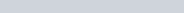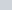# SSC CHSL Tier 1 Practice Set (English)

### Section 2: Reasoning : SSC CHSL Tier 1 Practice Set

Q.1: Select the option in which the numbers are related in the same way as are the numbers of the following set.
28 : 50 : 94
(A) 22 : 37 : 67
(B) 41 : 77 : 149
(C) 19 : 40 : 82
(D) 36 : 66 : 126

Ans : (D) 36 : 66 : 126

Q.2: Select the number from among the given options that can replace the question mark (?) in the following series.
8, 8, 11, 11, 14, 14, ?
(A) 22
(B) 14
(C) 18
(D) 17

Ans : (D) 17

Q.3: Find the number of triangle in the given figure.

(A) 26
(B) 22
(C) 24
(D) 34

Ans : (D) 34

Q.4: Read the given statements and conclusions carefully. Assuming that the information given in the statements is true, even if it appears to be at variance with commonly known facts, decide which of the given conclusions logically follow(s) from the statements.
Statements:
All rats are cats.
Some cats are not dogs.
Conclusions:
I. Some dogs are not rats.
II. Some dogs are rats.
(A) Both conclusions I and II follow.
(B) Only conclusions II follow.
(C) Only conclusions I follow.
(D) Neither conclusions I nor II follow.

Ans : (D) Neither conclusions I nor II follow

Q.5: Select the word-pair in which the two words are related in the same way as are the two words in the given word-pair.
Mathematics : Teacher
(A) Hockey : Coach
(B) Science : Researcher
(C) Student : Tutor
(D) Story : Book

Ans : (A) Hockey : Coach

Q.6: Select the figure that will come next in the following figure series.

Ans : a.

Q.7: Select the combination of letters that when sequentially placed in the blanks of the given series will complete the series.
s _ t m s r _ m _ r t _ s _ t m
(A) r, t, s, m, r
(B) r, t, r, m, s
(C) r, s, m, r, t
(D) r, s, t, r, m

Ans : (A) r, t, s, m, r

Q.8: Three different positions of the same dice are shown. Which symbol is on the face opposite to the face having the symbol ‘!’?

(A) &
(B) %
(C) #
(D) \$

Ans : (A) &

Q.9: Four words have been given, out of which three are alike in some manner and one is different. Select the word that is different.
(A) Steel
(B) Brass
(C) Nickel
(D) Bronze

Ans : (C) Nickel

Q.10: The cost of 5 pens and 12 pencils is 45. what would be the cost for 20 pens and 48 pencils?
(A) 180
(B) 120
(C) 135
(D) 235

Ans : (A) 180

Q.11: Select the set in which the numbers are related in the same way as are the numbers of the following set.
(313, 2732, 98211)
(A) (103, 7341, 92501)
(B) (601, 9401, 71427)
(C) (201, 4532, 65712)
(D) (301, 6543, 94263)

Ans : (B) (601, 9401, 71427)

Q.12: Which of the following numbers does NOT belong to the group?
423, 171, 801, 900, 701
(A) 171
(B) 701
(C) 801
(D) 423

Ans : (B) 701

Q.13: Select the option that is embedded in the given figure (rotation is NOT allowed).

Ans : a.

Q.14: Three of the following four letter clusters are alike in a certain way and one is different. Select the odd one.
(A) YTSR
(B) AEKM
(C) IMPV
(D) JMSU

Ans : (A) YTSR

Q.15: Select the option that is related to the third number in the same way as the second number is related to the first number.
166 : 412 : : 256 : ?
(A) 640
(B) 549
(C) 428
(D) 597

Ans : (A) 640

Q.16: The sequence of folding a piece of paper and the manner in which the folded paper has been cut are shown in the following figure. How would this paper look when unfolded?

Ans : b.

Q.17: M’s wife is N’s daughter-in-law. P is the husband of o, who is the sister of M. How is P related to N ?
(A) Brother
(B) Cousin
(C) Nephew
(D) Son-in-law

Ans : (D) Son-in-law

Q.18: If in a code language, ORGAN is coded as 17141518 and FINGER is coded as 56791418, then how will you code NECK?
(A) 113497
(B) 248741
(C) 351114
(D) 532761

Ans : (C) 351114

Q.19: In a certain code language, HUSBAND is written as ABDHNSU. How will HOUSEWIFE be written in that language ?
(A) EFIWESUOH
(B) EHEFOIUWS
(C) EEFHIOSUW
(D) ESEFUOIWH

Ans : (C) EEFHIOSUW

Q.20: Select the correct mirror image of the given figure when the mirror is placed at the right side.

Ans : b.

Q.21: which two digits and signs need to be interchanged so as to balance the given equation ?$75-13+19\times8\div5=93$
(A) 7 and 9; + and x
(B) 5 and 8; – and$\div$
(C) 3 and 5; – and x
(D) 8 and 3; x and$\div$

Ans : (B) 5 and 8; – and$\div$

Q.22: Select the option that is related to the third term in the same way as the second term is related to the first term.
SHORTLY : OHSYLTR : : WALKING : ?
(A) LAWNIKG
(B) KLAWGNI
(C) AWKLNIG
(D) LAWGNIK

Ans : (D) LAWGNIK

Q.23: Select the option that is related to the third word in the same way as the second word is related to the first word.
Wakeful : Sleepless : : Sitting : ?
(A) Idle Resting
(B) Situated
(C) Sedentary

Ans : (C) Sedentary

Q.24: Arrange the following words in a meaningful sequence.
1. Manager
2. Worker
3. General manager
4. Supervisor
5. Owner
(A) 3, 5, 4, 1, 2
(B) 1, 3, 5, 2, 4
(C) 4, 3, 2, 1, 5
(D) 5, 3, 1, 4, 2